# Straight lines

Draw two lines c, d so that c || d. On line c mark points A, B, from point A start perpendicular to line c, from point B perpendicular to line c.

Leave us a comment of example and its solution (i.e. if it is still somewhat unclear...):

Showing 1 comment:Math student
I dont knw hw to calculate this## Next similar examples:

1. Neighbor angle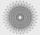For 136° angle calculate size of adjacent angle on one side of a straight line.
2. Draw it!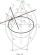Draw two lines c, d that c || d. On line c mark the points A, B. By point A lead perpendicular line to c. By point B lead perpendicular line to c.
3. Three points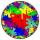Mark three points E, F and G in the plane not lie on one line. a) Draw a line segment FG b) Construct halfline (ray) EG c) Draw a line EF
4. Square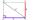Draw a square on the edge of a = 4 cm. Mark the center of symmetry S and all axes of symmetry. How many axes of symmetry does? Write down.
5. RectangleDraw a rectangle with the sides a = 4 cm, b = 5 cm. Mark the center of symmetry S and all axes of symmetry. How many axes of symmetry has? Write down.
6. The sum graphically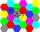Draw a graphically sum of the all sides of 4-gon ABCD.
7. Angle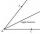Draw angle |∠ ABC| = 130° and built its axis. What angle is between axis angle and arm of angle?
8. Line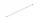How many parts of line divide 5 (different) points that lie on it?
9. Tangents constructCircle is given k (S; 2.5 cm) and an outer line p. Construct a tangent t of the circle that has with a line p angle 60°. How many solutions has the task?
10. Plane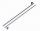On how many parts divide plane 6 parallels?
11. SlopeFind the slope of the line: x=t and y=1+t.
12. Angles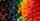Which of those angles are obtuse?
13. Obtuse angleWhich obtuse angle is creating clocks at 17:00?
14. Compare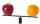Compare with characters >, <, =: 85.57 ? 80.83
15. Roman numerals +Add up the number writtens in Roman numerals. Write the results as a decimal number.
16. TriangleProve whether you can construct a triangle ABC, if a=9 cm, b=10 cm, c=4 cm.
17. To thousands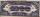Round to thousands following numbers: